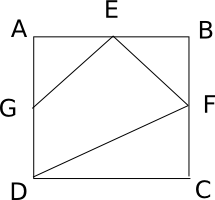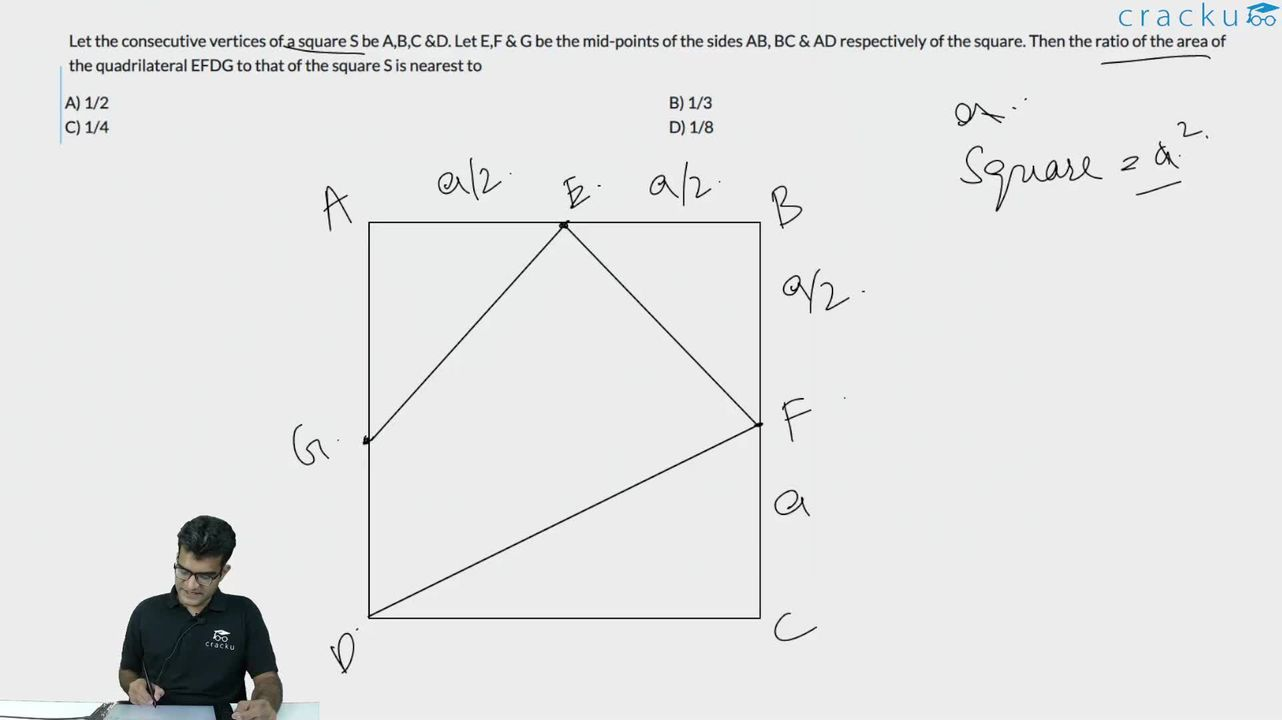Question 20

# Let the consecutive vertices of a square S be A,B,C &D. Let E,F & G be the mid-points of the sides AB, BC & AD respectively of the square. Then the ratio of the area of the quadrilateral EFDG to that of the square S is nearest to

SolutionLet the side of the square be 1 cm

So, area of triangle AGE = 1/2 * 1/2 * 1/2 = 1/8

Similarly, area of triangle EBF = 1/8

Area of triangle DFC = 1/2 * 1 * 1/2 = 1/4

Area of the square = 1*1 = 1

So, area of the quadrilateral = 1 - (1/8 + 1/8 + 1/4) = 1 - 1/2 = 1/2

Option a) is the correct answer.

### View Video Solution